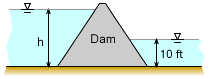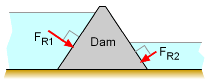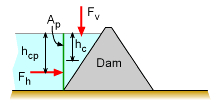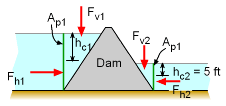Ch 2. Fluid Statics Multimedia Engineering Fluids PressureVariation PressureMeasurement HydrostaticForce(Plane) HydrostaticForce(Curved) Buoyancy
 Chapter 1. Basics 2. Fluid Statics 3. Kinematics 4. Laws (Integral) 5. Laws (Diff.) 6. Modeling/Similitude 7. Inviscid 8. Viscous 9. External Flow 10. Open-Channel Appendix Basic Math Units Basic Equations Water/Air Tables Sections Search eBooks Dynamics Fluids Math Mechanics Statics Thermodynamics Author(s): Chean Chin Ngo Kurt Gramoll ©Kurt GramollFLUID MECHANICS - EXAMPLE QuestionsA Gravity Dam A gravity dam can resist a maximum lateral force of Fm = 78, 000 lb. (a) What is the maximum height of the dam if there is no downstream (right side of dam)? (b) What if the water depth downstream is 10 ft? (c) Would it be a good idea to design the dam for the condition of part (b)? SolutionThe Resultant Forces Act Perpendicular to the DamThe Lateral Force Component on only One Side of Dam Ap (a) The resultant force (FR) due to water pressure is perpendicular to the dam surface. Here, only concerned with lateral (horizontal) component, so you can use the projected vertical dimension of the dam. The lateral force (FH) due to fluid pressure (hydrostatic conditions) acting on the projected area Ap can be determined for a unit width as      Fh = γ hc Ap = γ (h/2) Ap where h is the total water depth. To find the maximum h, set Fh to the allowable force, Fm, to give      Fh = Fm      (62.4 lb/ft3) (h/2) [h (1 ft)] = 78,000 lb      h = [78,000 / 31.2]0.5 = 50 ftLateral Force Components Acting on the Dam (b) If the water depth downstream is 10 ft, then a resultant force will act on that side of the dam, and will counteract the upstream force.      Fh1 - Fh2 = Fm      γ hc1 Ap1- γ hc2 Ap2 = 78,000 lb      62.4 (h/2) [(h)(1)] - 62.4 (5) [(10)(1)] = 78,000      31.2 h2 = 81,120     h = 51.0 ft (c) Since the 10 ft of water downstream may not exist in times of draught, the dam should be limited to 50 ft of water.

Practice Homework and Test problems now available in the 'Eng Fluids' mobile app
Includes over 250 problems with complete detailed solutions.
Available now at the Google Play Store and Apple App Store.